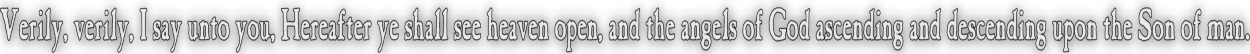None:
Polyps:
Strongs:MetaphysicsAuthority For The Sons Of God However the terms in Ay(x) would indicate that if both x and y hold L(G) then they are both redeemable, but the operator "A" is such as to enable 'y' to redeem 'x' if 'x' is at fault. This would be to ensure that L(G) is held correctly: i.e. Y may be substituted for y with Y = HG(y) We state that in order for y to be able to correct 'x', the union of redeemed values in Y = HG(y) with those values in Hx(x) are redeemable to HG(HG(y) ∪ Hx(x)) = HG(Y ∪ Hx(x)). This set contains every correct virtue held by x and y, without permitting any faults of virtue by x to persist, and also contains every positive property or virtue known by y. It must also contain L(G), as we considered only the redeemed set Y. So in order for x to be fully corrected, we assume Hx(x) is now redeemed to HG(Y ∪ Hx(x)). So what are the implications? If Y is substituted for y, then the set of correct beliefs of y (held with L(G)) in Y = HG(y) may be so as to refine those held by Hx(x) to HG(x). Clearly HY(x) are correct if x holds to L(G) also. However Ay(x) would allow 'y' with authority to correct 'x' if 'x' were not to hold to L(G). Then we see that "Ay(x)" is a forceful operator simply dividing those with "divine authority" to correct others from those without. There is one caveat however, that 'y' may be correct in virtue yet still not hold to L(G). Then, for a redeemable 'x' at fault in contradicting some virtue yet holding L(G), we may perceive that Ay(x) could actually be true whereas Ax(y) is untrue. However this is never the case! For 'x' must be redeemed if he holds L(G) and 'y' is not redeemed; and not even HG(G) \ L(G) = HG(y) will necessarily justify a man before God, (if 'y' could claim to be perfect). x is also necessarily "not God". To have the authority 'x' must have been redeemed rather than merely redeemable: the operator Ax(y) makes no "sense". We would be able to state in this case that 'y' would have invented his own laws somewhat in common with God's own but not in completeness - 'x' though temporarily in fault presently has no "authority" to correct y. Rather than justify hypocrisy we should simply state that "redemption is more important that fault": for if HG(G) \ L(G) does not justify, neither will HG(y) (a subset of the former) which is without L(G). (All have sinned and fall short of the glory of God.) Then all we should note from this for now is that Ay(x) is true if 'y' is redeemed and 'x' is at fault or not. We may substitute Y = HG(y) for y and Hx(x) for x in Ay(x) without issue. Likewise we would state that Ay(x) is true only if y is an "l" from the set of chosen elect - the saved. Thus from the statement regarding hypocrisy above, we note that L(G) if held by an individual indicates salvation as well as the authority to correct any such x, since HG(G) \ L(G) is also a fault! L(G) implies authority "from God" down over His creations to the individual y. We note from the universality of AG(y), that HG(G) = HG(y) may hold only if y holds L(G). Rather than put y=G, we simply state that AG(y) - we require the possibility for y to become virtuous at least. Then L(G) => AG(y) universally. So then, given G "God", then AG(G)<=>L(G) i.e. God is indeed without fault. Therefore in the model Ay(x) is well defined for all y holding L(G). I.e. we may substitute AG(x) for Ay(x) if and only if y holds L(G). y holds L(G) => y holds AG(G) => y holds AG(y) =>Hy(y) is redeemed to HY(y) => Y is a correct set of virtues with L(G) => HY(y) is a correct set of virtues => HY(y) "may correct" Hx(x) Hx(x) becomes at best HG(HY(y) union Hx(x)) Which must contain L(G) (y must have been redeemed to Y) Hx(x) now holds a proper set of virtues in HG(G) with L(G) whether x previously held L(G) or not. In the case that the resultant Hx(x) does not then contain L(G) we must have that 'y' did not hold L(G); rather we consider the case Y when y is redeemed in full accord with L(G)<=>AG(G) and AG(y). We would assert that the "authority exists" to correct x. And this authority is ultimately equal to AG(G)<=>L(G) which is over all 'x' and 'y' to correct members in a chain in 'y' down to 'x'. Thus we may indeed substitute AG(x) for Ay(x) if y holds true to L(G). Thus the authority for a believer to correct some other's virtues may then be deemed to come directly from God. The "authority" depends fully on the "correctness" one ascribes to the laws of God. Right and wrong are concepts that spring from our virtues, and as we are made in the image of God we would state that virtues are persistent and all men are able to be persuaded as to them: Correcting each others faults (the speck) in the breaking of commandments is then reliant completely on whether an individual is redeemed in the gospel and has removed the "beam from their own eye" first. Continue To Next Page Return To Section Start Return To Previous Page'